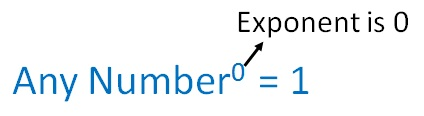If power is 0, anwser is always 1

2 0 = 1

3 0 = 1

1000 0 = 1

How did we get it?

We write

2 0 = 2 1 − 1

= 2 1 × 2 −1

= 2 × 1/2

= 2/2

= 1

Similarly, we can prove this for any number

1. Chapter 12 Class 8 Exponents and Powers
2. Concept wise
3. Exponents

Exponents